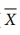# Summary

In this chapter(Applied Statistics) we have acquired the knowledge of

Summary

In this chapter we have acquired the knowledge of

Method of Moving Averages

Three year moving averages:Four year moving averages:Method of Least Squares

The straight line equation, Y = a + bX

Two Normal Equations, ΣY = n a + b ΣX ; ΣXY = a ΣX + b ΣX2

Methods of measuring Seasonal Variations-Method of Simple Averages:

Seasonal Index (S.I) = Seasonal Average/ Grand average  X100

If the data is given in months

S.I for Jan = Monthly Average ( for Jan)/ Grand average  x 100

If the data is given in quarter

S.I for Kth Quarter = Average of Kth quarter/Grand average X  100

Continuous distribution function

If X is a continuous random variable with the probability density function f X (x), then the function FX (x) is defined by

Weighted Index Number

Price Index (P01), Laspeyre’s price index number, Paasche’s price index number, Fisher’s price index numberTime Reversal Test :Cost of Living Index Number

Aggregate Expenditure Method =Family Budget Method = ∑ PV / ∑ V

Causes of Variation

1. Chance Causes (or) Random causes 2. Assignable Causes

Control Charts

(i) Centre Line (CL) indicates the desired standard level of the process.

(ii) Upper Control Limit (UCL) indicates the upper limit of tolerance.

(iii) Lower Control Limit (LCL) indicates the lower limit of tolerance.

(i) Centre Line (CL) indicates the desired standard level of the process.

(ii) Upper Control Limit (UCL) indicates the upper limit of tolerance.

(iii) Lower Control Limit (LCL) indicates the lower limit of tolerance.

The control limits forchart in two different cases areThe control limits for R chart in two different cases areTags : Applied Statistics , 12th Business Maths and Statistics : Chapter 9 : Applied Statistics
Study Material, Lecturing Notes, Assignment, Reference, Wiki description explanation, brief detail
12th Business Maths and Statistics : Chapter 9 : Applied Statistics : Summary | Applied Statistics